# Raman Spectroscopy

## Theoretical background​

Raman spectroscopy is an analytical technique mainly used to determine the vibrational modes of molecules. The working principle of such technique relies on a monochromatic light (e.g. visible, near infrared or near ultraviolet) which is shined on a sample and excites the molecule to a virtual energy state for a short time. The emitted photons can present either higher or lower energy than the incident photon and this change in the kinetic energy is known as inelastic scattering which is specific to a molecule's chemical bond. Raman spectroscopy is often used in chemistry in order to indentify molecules and assessing the chemical bonds present in the molecule.

The induced dipole moment of a molecule due to an external electric field can be written as,

$\mathbf{\mu}_\text{induced} = \overleftrightarrow \alpha \mathbf{E}$

Where $\overleftrightarrow \alpha$ is the polarizability tensor and $\mathbf{E}$ is the external electric field. The polarizability tensor can be represent by a 2x2 matrix as following

$\overleftrightarrow \alpha = \begin{bmatrix} \alpha_{xx} & \alpha_{xy} & \alpha_{xz}\\ \alpha_{yx} & \alpha_{yy} & \alpha_{yz}\\ \alpha_{zx} & \alpha_{zy} & \alpha_{zz} \end{bmatrix}$

### Selection rules​

If the applied electric field is constant, the intensity of a transition is proportional the the integral:

$\int \psi_{2 \text{vib}}^* \hat{\mu} \psi_{1 \text{vib}} \mathrm{d} Q \propto \int \psi_{2 \text{vib}}^* \hat{\alpha}_{ij} \psi_{1 \text{vib}} \mathrm{d} Q$

Where $\psi_{2 \text{vib}}$ is the upper level vibrational wavefunction and $\psi_{1 \text{vib}}$ the lower one.

These kind of integrals can be estimated in two ways: either by group theory or by an approximation.

Using group theory, the integral is non zero if and only if the direct product of the integrand contains the totally symmetric representation, meaning that,

$\Gamma (\psi_{2 \text{vib}}) \otimes \Gamma (\hat{\alpha}_{ij}) \otimes \Gamma (\psi_{2 \text{vib}}) \subset \Gamma_{\text{tot sym}}$

The other method consists of expanding one component of the polarizability tensor as a power seres in the normal coordinates $Q_k$:

$\alpha_{ij} = (\alpha_{ij})_0 + \sum_k \left(\frac{\partial \alpha_{ij} }{\partial Q_k} \right)_0 Q_k + \sum_k \sum_j \left(\frac{\partial^2 \alpha_{ij} }{\partial Q_k \partial Q_j} \right)_0 Q_k Q_j + ...$

If we take only the first two terms and we neglect higher order terms, this lead to the integral, for a single particle,

$\int \psi_{2 \text{vib}}^* \hat{\alpha}_{ij} \psi_{1 \text{vib}} \mathrm{d} Q_k = (\alpha_{ij})_0 \int \psi_{2 \text{vib}}^* \psi_{1 \text{vib}} \mathrm{d} Q_k + \left(\frac{\partial \alpha_{ij} }{\partial Q_k} \right)_0 \int \psi_{2 \text{vib}}^* Q_k \psi_{1 \text{vib}} \mathrm{d} Q_k$

Therefore, the Raman vibrational transition is allowed for a change in vibrational quantum number of $\pm 1$ ($\Delta v = \pm 1$).

# Raman spectra

You can upload a JCAMP or a txt file in the following way: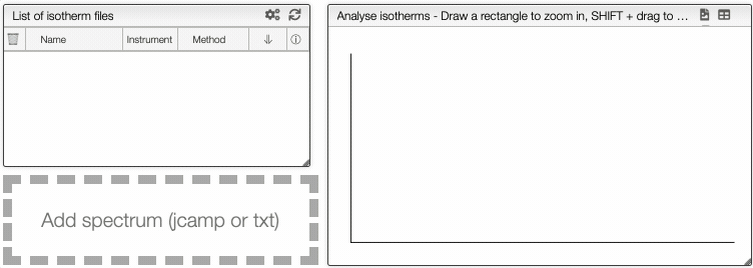## Preprocessing​

At the right of the graph, you can apply filters on the curve using the preprocessing module.

Spectrum preprocessing
Apply mathematical tools to the spectra.

## Preprocessing​

You can apply the following modifications to the spectra to enhance the visualization. The modifications include the following:

• Center Mean : subtract the mean from every variable observation in the dataset, so that the new variable's mean is centered at 0.
• Divide by SD : divide every variable observable in the dataset by the standard deviation yields a distribution with a standard deviation equal to 1.
• Divide by max Y : divide every value by the maximum y-value shifts all the y-values between 0 and 1.
• Rescale (x to y) : rescale the graph such that the y-values fit between specified minimum and maximum values.
• Normalize (sum to n) : normalize the integral under the curve so that it sums to n.
• Multiply (value) : multiply every y-value by a scalar.
• Add (value) : add a scalar to every y-value.
• AirPLS baseline : baseline correction using adaptive iterative reweighed penalized least squares algorithm.
• Rolling average baseline :
• Iterative polynomial baseline : baseline correction using iterative polynomial fitting algorithm.
• Rolling ball baseline :
• Rolling median baseline :

A certain range of x-values can be selected to show only a part of the spectrum using Range.

Depending on the analysis, some regions should be removed using Exclusions in order to improve the visualization.## Peak picking​

Bellow the preprocessing module, it is possible to manually pick peaks on a spectrum by holding down the ALT key, and then left-clicking on the peaks of interest. Or, you can select the interval that you want and click on Auto peak picking to choose the picks of interest automatically.

Manual peak picking
Informations about the manual peak picking.

## Peak picking​

It is possible to manually pick peaks in a spectrum by holding down the ALT key, and then left-clicking on the peaks of interest.

The application will systematically look for the strongest signal close to the wavelength that has been clicked. The peaks will be stored in a separate tab.

The algorithm automatically classifies the peaks.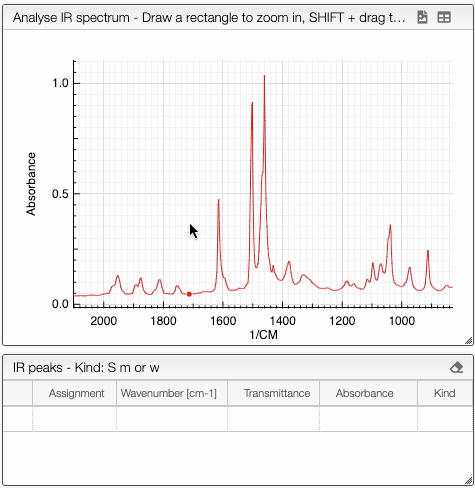Auto peak picking
Informations about the auto peak picking.

## Auto peak picking​

The system can automatically pick peaks of interest for a specific range of wavenumbers with a defined noise level using Auto peak picking tab.

Auto peak picking is based on the Savitzky Golay filter.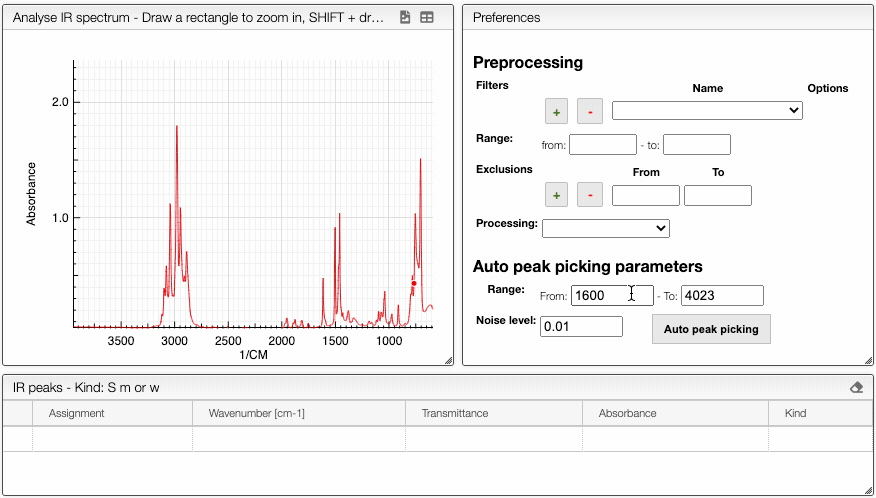caution

The auto peak picking is processed before the application of filters. If the spectra has too much noise, it is better to use manual peak picking

## Export​

You can export the spectrum as a svg or a pdf file. You can also export in two different formats the results of the peak picking.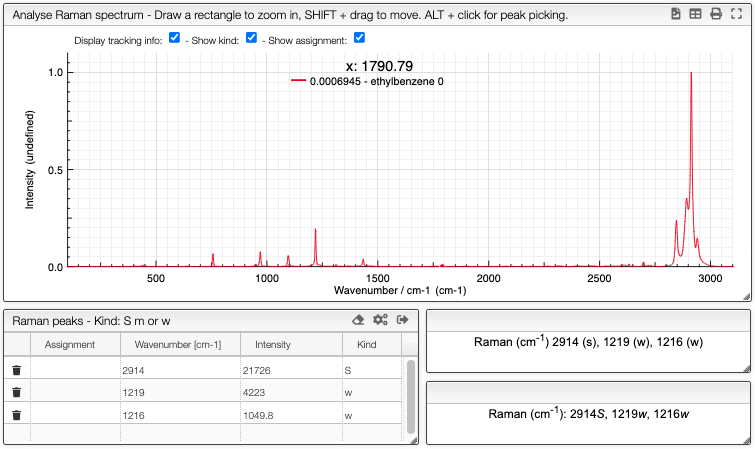## Compare spectra​

You can compare multiple Raman spectra by first opening up a spectrum, and then clicking Compare on top of graph.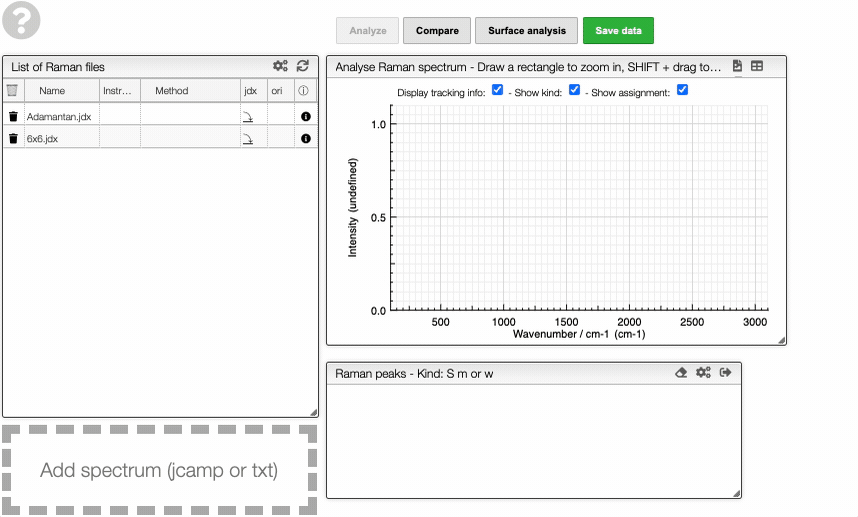The spectra will then be both displayed on top of each other. You can also freely hide selected graphs and change the color by clicking the color bar.

Visualising spectra
How to show/hide spectra.

## Visualising spectra​

If you wish to see only certain spectra, it is possible to selectively hide (or delete) them.

In order to do so, use the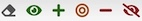buttons on the top panel of the displayed spectra list.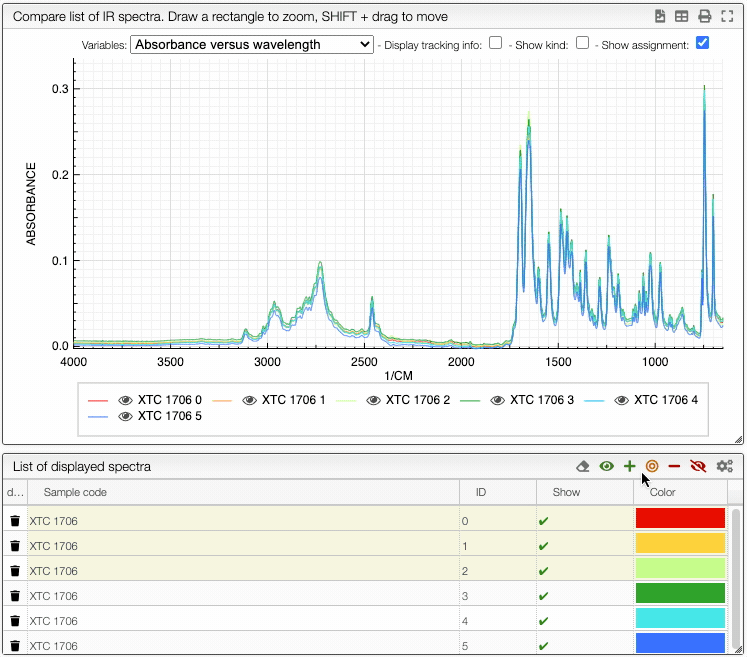You can also change the color of an individual spectrum in the displayed spectra tab with a double click.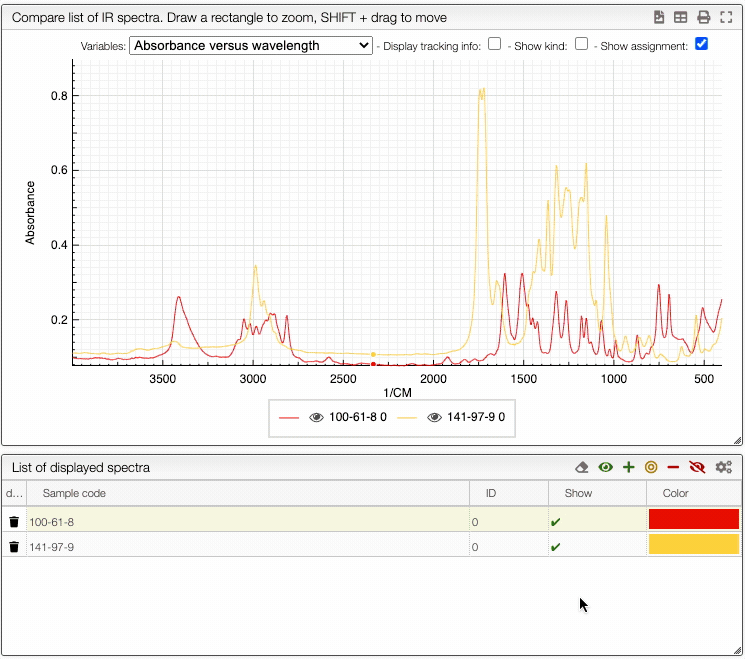## Surface analysis​

If the graph contains the data, you can click on Surface analysis. In the Raman image, you can go on each pixel with the cursor and you will see the corresponding spectrum on the right. If you click on one pixel and put the cursor on another, you will be able to compare the two spectrum, the one that you click will appear in red and the curve of the pixel under the cursor will be blue.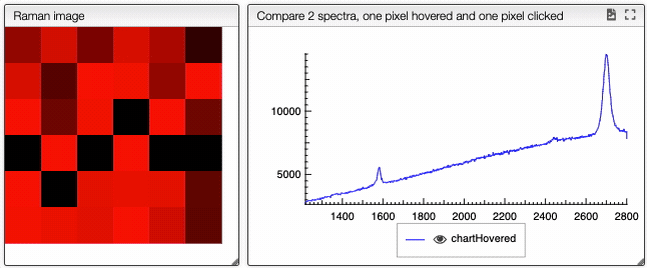On the bottom, you have the preprocessing command to apply filters on all the spectra. Spectra are plotted all in the same graph, at the bottom right. With the Image color module, you can rescale the pixels to a specific color proportionally to its intensity on its spectrum.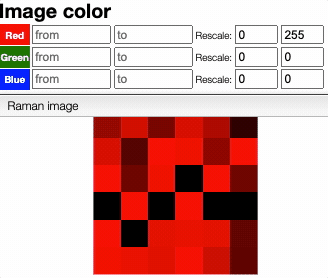In the graph containing all the spectra, you can choose to show the original data or the normalized spectra. If the spectra are normalized, you can add box plot to all curve so that we see the median of the distributions and the trends.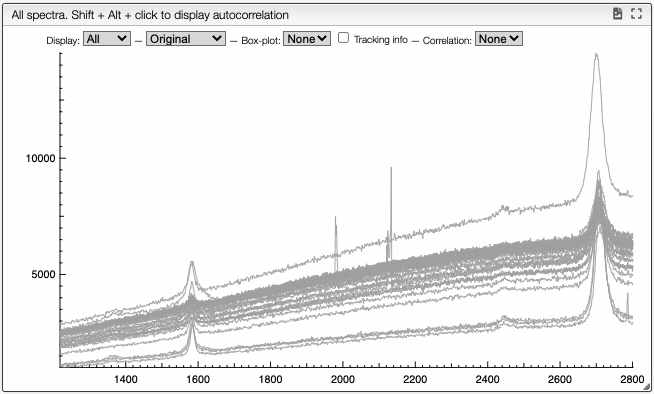Additionally, you can activate the correlation. Correlation is explained bellow. Here, you can either select all the spectra to be correlated or none of them.

info
Correlation of the graph.

## Correlation​

The correlation is a tool used to determine the similarity between a set of curves. It is a measure of how the intensity of a peak vary with the intensity of another peak. It allows us to isololate side product for example.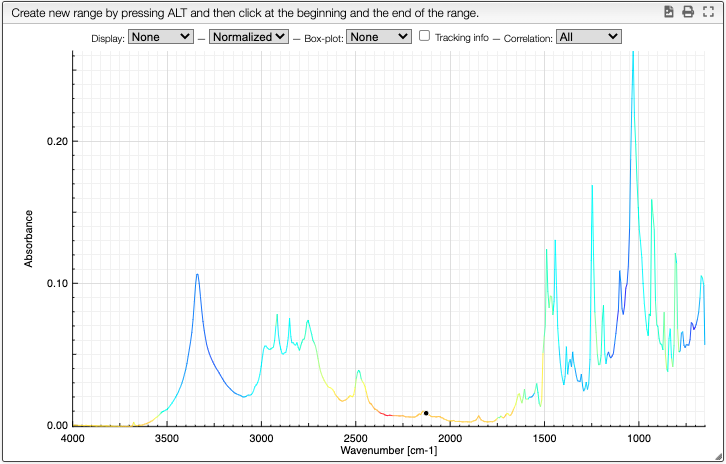Columns customization
In many tables it is possible to select which columns to display. This is achieved by clicking on the icon.

After clicking on the icon a dialog box opens that allows to add a new column.

There are 5 parameters to fill for a new column:

• name: the column name. This will be displayed as the header to the column.
• rendererOptions: options that allows among other to format numbers. One very useful formatter is numeral (see after)
• width: number of pixels for the specific column. May stay empty for automatic layout.
• forceType: select how to display complex values (see later)
• jpath: where to find the information to display in the column

In order to add a new column you need to select the jpath using the hierarchical drop down menu.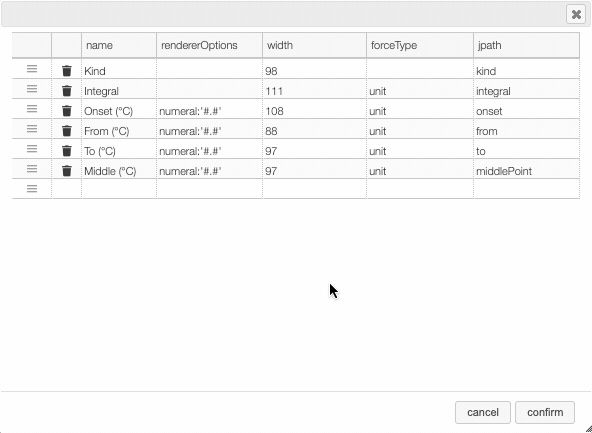Columns can be moved or rearranged as well.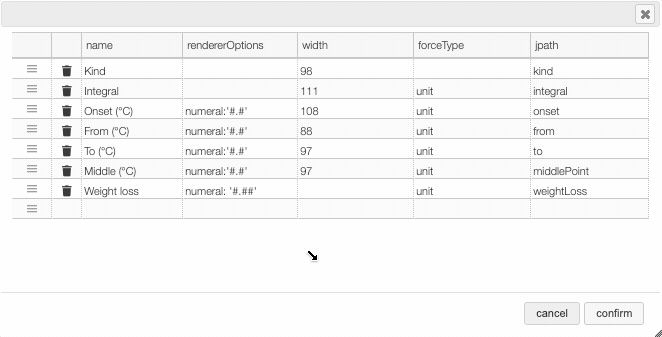rendererOptions: numeral

number,

Value to formatrendererOptionsResult
12.345678numeral:'#.##'12.34
12.3numeral:'#.00'12.30
0.3numeral:'#.0 %'30.0 %

forceType

In the database some values are stored as an object that needs to be displayed to the user in an intuitive way.

For example the unit type will store in the database the value using as units SI (we convert the data to the units defined by the 'standard international') and specify in which units the user would like to display the data. So in the following example we are in fact storing the value -150°C by storing the value in SI (Kelvin) and specifying that the user wants to see it in °C.

{  "SI": 123.15,  "unit": "°C"}

Another example is the valueUnits type that will store the data in 2 different properties (value and unit). In this case the value is stored in the specified units and there is no conversions.

{  "value": 123,  "units": "°C"}

While this way to store the data in the database is very practical it is not the way that the user would like to see his results. We have therefore the possibility to forceType: define how the user would like to see the results.

Other types include mf. This formatter allows to correctly display a molecular formula that is stored in the database as "C10H20O3". i.e. it will put the numbers in subscript (C₁₀H₂₀O₃).

1. M. Drabbels, Lectures Notes "Spectroscopy", EPFL, 2022.